## Matplotlib 2.0.0rc2 is available

Install the release candidate now!Travis-CI:# Annotating Axes¶

Do not proceed unless you already have read Annotating text, `text()` and `annotate()`!

## Annotating with Text with Box¶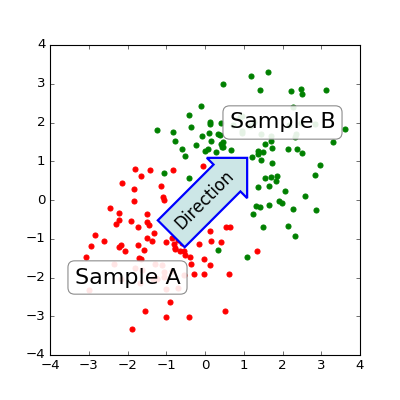The `text()` function in the pyplot module (or text method of the Axes class) takes bbox keyword argument, and when given, a box around the text is drawn.

```bbox_props = dict(boxstyle="rarrow,pad=0.3", fc="cyan", ec="b", lw=2)
t = ax.text(0, 0, "Direction", ha="center", va="center", rotation=45,
size=15,
bbox=bbox_props)
```

The patch object associated with the text can be accessed by:

```bb = t.get_bbox_patch()
```

The return value is an instance of FancyBboxPatch and the patch properties like facecolor, edgewidth, etc. can be accessed and modified as usual. To change the shape of the box, use set_boxstyle method.

```bb.set_boxstyle("rarrow", pad=0.6)
```

The arguments are the name of the box style with its attributes as keyword arguments. Currently, following box styles are implemented.

Class Name Attrs
Circle `circle` pad=0.3
DArrow `darrow` pad=0.3
LArrow `larrow` pad=0.3
RArrow `rarrow` pad=0.3
Round `round` pad=0.3,rounding_size=None
Round4 `round4` pad=0.3,rounding_size=None
Roundtooth `roundtooth` pad=0.3,tooth_size=None
Sawtooth `sawtooth` pad=0.3,tooth_size=None
Square `square` pad=0.3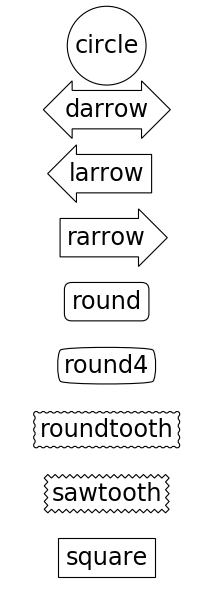Note that the attributes arguments can be specified within the style name with separating comma (this form can be used as “boxstyle” value of bbox argument when initializing the text instance)

```bb.set_boxstyle("rarrow,pad=0.6")
```

## Annotating with Arrow¶

The `annotate()` function in the pyplot module (or annotate method of the Axes class) is used to draw an arrow connecting two points on the plot.

```ax.annotate("Annotation",
xy=(x1, y1), xycoords='data',
xytext=(x2, y2), textcoords='offset points',
)
```

This annotates a point at `xy` in the given coordinate (`xycoords`) with the text at `xytext` given in `textcoords`. Often, the annotated point is specified in the data coordinate and the annotating text in offset points. See `annotate()` for available coordinate systems.

An arrow connecting two point (xy & xytext) can be optionally drawn by specifying the `arrowprops` argument. To draw only an arrow, use empty string as the first argument.

```ax.annotate("",
xy=(0.2, 0.2), xycoords='data',
xytext=(0.8, 0.8), textcoords='data',
arrowprops=dict(arrowstyle="->",
connectionstyle="arc3"),
)
```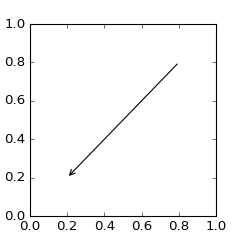The arrow drawing takes a few steps.

1. a connecting path between two points are created. This is controlled by `connectionstyle` key value.
2. If patch object is given (patchA & patchB), the path is clipped to avoid the patch.
3. The path is further shrunk by given amount of pixels (shirnkA & shrinkB)
4. The path is transmuted to arrow patch, which is controlled by the `arrowstyle` key value.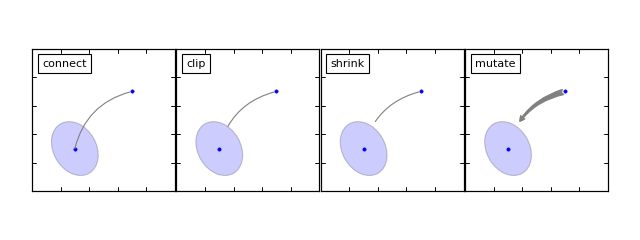The creation of the connecting path between two points is controlled by `connectionstyle` key and following styles are available.

Name Attrs
`angle` angleA=90,angleB=0,rad=0.0
`angle3` angleA=90,angleB=0
`arc` angleA=0,angleB=0,armA=None,armB=None,rad=0.0
`arc3` rad=0.0
`bar` armA=0.0,armB=0.0,fraction=0.3,angle=None

Note that “3” in `angle3` and `arc3` is meant to indicate that the resulting path is a quadratic spline segment (three control points). As will be discussed below, some arrow style option only can be used when the connecting path is a quadratic spline.

The behavior of each connection style is (limitedly) demonstrated in the example below. (Warning : The behavior of the `bar` style is currently not well defined, it may be changed in the future).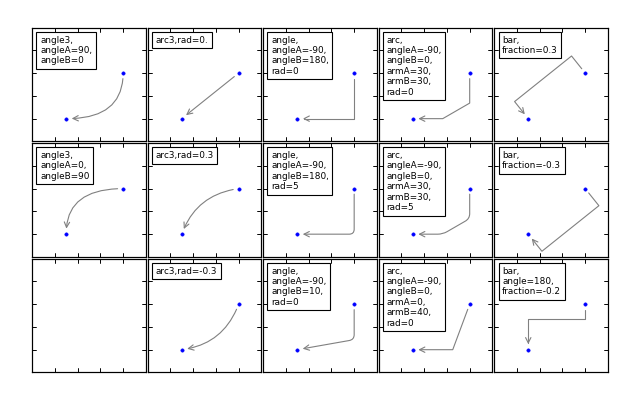The connecting path (after clipping and shrinking) is then mutated to an arrow patch, according to the given `arrowstyle`.

Name Attrs
`-` None
`->` head_length=0.4,head_width=0.2
`-[` widthB=1.0,lengthB=0.2,angleB=None
`|-|` widthA=1.0,widthB=1.0
`-|>` head_length=0.4,head_width=0.2
`<-` head_length=0.4,head_width=0.2
`<->` head_length=0.4,head_width=0.2
`<|-` head_length=0.4,head_width=0.2
`<|-|>` head_length=0.4,head_width=0.2
`fancy` head_length=0.4,head_width=0.4,tail_width=0.4
`simple` head_length=0.5,head_width=0.5,tail_width=0.2
`wedge` tail_width=0.3,shrink_factor=0.5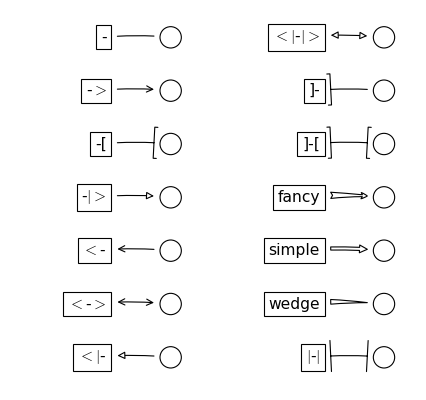Some arrowstyles only work with connection style that generates a quadratic-spline segment. They are `fancy`, `simple`, and `wedge`. For these arrow styles, you must use “angle3” or “arc3” connection style.

If the annotation string is given, the patchA is set to the bbox patch of the text by default.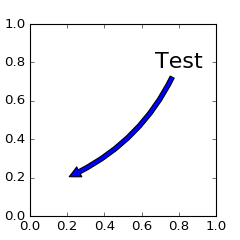As in the text command, a box around the text can be drawn using the `bbox` argument.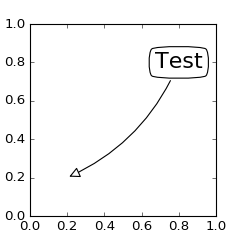By default, the starting point is set to the center of the text extent. This can be adjusted with `relpos` key value. The values are normalized to the extent of the text. For example, (0,0) means lower-left corner and (1,1) means top-right.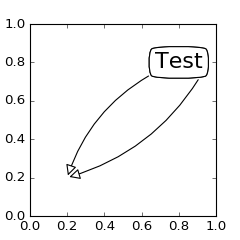## Placing Artist at the anchored location of the Axes¶

There are class of artist that can be placed at the anchored location of the Axes. A common example is the legend. This type of artists can be created by using the OffsetBox class. A few predefined classes are available in `mpl_toolkits.axes_grid.anchored_artists`.

```from mpl_toolkits.axes_grid.anchored_artists import AnchoredText
at = AnchoredText("Figure 1a",
prop=dict(size=8), frameon=True,
loc=2,
)
```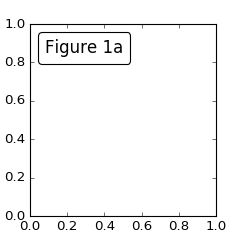The loc keyword has same meaning as in the legend command.

A simple application is when the size of the artist (or collection of artists) is known in pixel size during the time of creation. For example, If you want to draw a circle with fixed size of 20 pixel x 20 pixel (radius = 10 pixel), you can utilize `AnchoredDrawingArea`. The instance is created with a size of the drawing area (in pixel). And user can add arbitrary artist to the drawing area. Note that the extents of the artists that are added to the drawing area has nothing to do with the placement of the drawing area itself. The initial size only matters.

```from mpl_toolkits.axes_grid.anchored_artists import AnchoredDrawingArea

ada = AnchoredDrawingArea(20, 20, 0, 0,
p1 = Circle((10, 10), 10)
p2 = Circle((30, 10), 5, fc="r")
```

The artists that are added to the drawing area should not have transform set (they will be overridden) and the dimension of those artists are interpreted as a pixel coordinate, i.e., the radius of the circles in above example are 10 pixel and 5 pixel, respectively.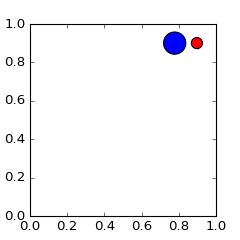Sometimes, you want to your artists scale with data coordinate (or other coordinate than canvas pixel). You can use `AnchoredAuxTransformBox` class. This is similar to `AnchoredDrawingArea` except that the extent of the artist is determined during the drawing time respecting the specified transform.

```from mpl_toolkits.axes_grid.anchored_artists import AnchoredAuxTransformBox

box = AnchoredAuxTransformBox(ax.transData, loc=2)
el = Ellipse((0,0), width=0.1, height=0.4, angle=30) # in data coordinates!
```

The ellipse in the above example will have width and height corresponds to 0.1 and 0.4 in data coordinate and will be automatically scaled when the view limits of the axes change.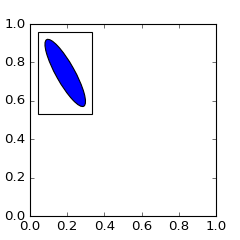As in the legend, the bbox_to_anchor argument can be set. Using the HPacker and VPacker, you can have an arrangement(?) of artist as in the legend (as a matter of fact, this is how the legend is created).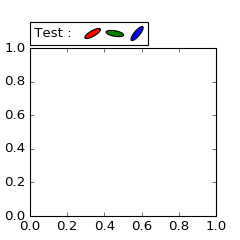Note that unlike the legend, the `bbox_transform` is set to IdentityTransform by default.

## Using Complex Coordinate with Annotation¶

The Annotation in matplotlib support several types of coordinate as described in Annotating text. For an advanced user who wants more control, it supports a few other options.

1. `Transform` instance. For example,

```ax.annotate("Test", xy=(0.5, 0.5), xycoords=ax.transAxes)
```

is identical to

```ax.annotate("Test", xy=(0.5, 0.5), xycoords="axes fraction")
```

With this, you can annotate a point in other axes.

```ax1, ax2 = subplot(121), subplot(122)
ax2.annotate("Test", xy=(0.5, 0.5), xycoords=ax1.transData,
xytext=(0.5, 0.5), textcoords=ax2.transData,
arrowprops=dict(arrowstyle="->"))
```
2. `Artist` instance. The xy value (or xytext) is interpreted as a fractional coordinate of the bbox (return value of get_window_extent) of the artist.

```an1 = ax.annotate("Test 1", xy=(0.5, 0.5), xycoords="data",
va="center", ha="center",
bbox=dict(boxstyle="round", fc="w"))
an2 = ax.annotate("Test 2", xy=(1, 0.5), xycoords=an1, # (1,0.5) of the an1's bbox
xytext=(30,0), textcoords="offset points",
va="center", ha="left",
bbox=dict(boxstyle="round", fc="w"),
arrowprops=dict(arrowstyle="->"))
```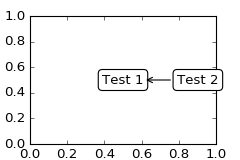Note that it is your responsibility that the extent of the coordinate artist (an1 in above example) is determined before an2 gets drawn. In most cases, it means that an2 needs to be drawn later than an1.

3. A callable object that returns an instance of either `BboxBase` or `Transform`. If a transform is returned, it is same as 1 and if bbox is returned, it is same as 2. The callable object should take a single argument of renderer instance. For example, following two commands give identical results

```an2 = ax.annotate("Test 2", xy=(1, 0.5), xycoords=an1,
xytext=(30,0), textcoords="offset points")
an2 = ax.annotate("Test 2", xy=(1, 0.5), xycoords=an1.get_window_extent,
xytext=(30,0), textcoords="offset points")
```
4. A tuple of two coordinate specification. The first item is for x-coordinate and the second is for y-coordinate. For example,

```annotate("Test", xy=(0.5, 1), xycoords=("data", "axes fraction"))
```

0.5 is in data coordinate, and 1 is in normalized axes coordinate. You may use an artist or transform as with a tuple. For example,

```import matplotlib.pyplot as plt

plt.figure(figsize=(3,2))
ax=plt.axes([0.1, 0.1, 0.8, 0.7])
an1 = ax.annotate("Test 1", xy=(0.5, 0.5), xycoords="data",
va="center", ha="center",
bbox=dict(boxstyle="round", fc="w"))

an2 = ax.annotate("Test 2", xy=(0.5, 1.), xycoords=an1,
xytext=(0.5,1.1), textcoords=(an1, "axes fraction"),
va="bottom", ha="center",
bbox=dict(boxstyle="round", fc="w"),
arrowprops=dict(arrowstyle="->"))
plt.show()
```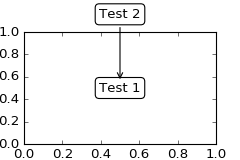5. Sometimes, you want your annotation with some “offset points”, but not from the annotated point but from other point. `OffsetFrom` is a helper class for such case.

```import matplotlib.pyplot as plt

plt.figure(figsize=(3,2))
ax=plt.axes([0.1, 0.1, 0.8, 0.7])
an1 = ax.annotate("Test 1", xy=(0.5, 0.5), xycoords="data",
va="center", ha="center",
bbox=dict(boxstyle="round", fc="w"))

from matplotlib.text import OffsetFrom
offset_from = OffsetFrom(an1, (0.5, 0))
an2 = ax.annotate("Test 2", xy=(0.1, 0.1), xycoords="data",
xytext=(0, -10), textcoords=offset_from,
# xytext is offset points from "xy=(0.5, 0), xycoords=an1"
va="top", ha="center",
bbox=dict(boxstyle="round", fc="w"),
arrowprops=dict(arrowstyle="->"))
plt.show()
```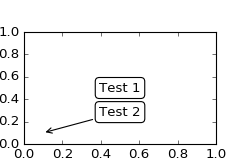You may take a look at this example pylab_examples example code: annotation_demo3.py.

## Using ConnectorPatch¶

The ConnectorPatch is like an annotation without a text. While the annotate function is recommended in most of situation, the ConnectorPatch is useful when you want to connect points in different axes.

```from matplotlib.patches import ConnectionPatch
xy = (0.2, 0.2)
con = ConnectionPatch(xyA=xy, xyB=xy, coordsA="data", coordsB="data",
axesA=ax1, axesB=ax2)
```

The above code connects point xy in data coordinate of `ax1` to point xy int data coordinate of `ax2`. Here is a simple example.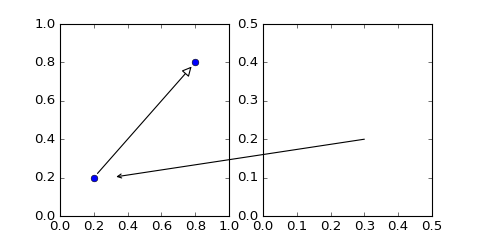While the ConnectorPatch instance can be added to any axes, but you may want it to be added to the axes in the latter (?) of the axes drawing order to prevent overlap (?) by other axes.

## Zoom effect between Axes¶

mpl_toolkits.axes_grid.inset_locator defines some patch classes useful for interconnect two axes. Understanding the code requires some knowledge of how mpl’s transform works. But, utilizing it will be straight forward.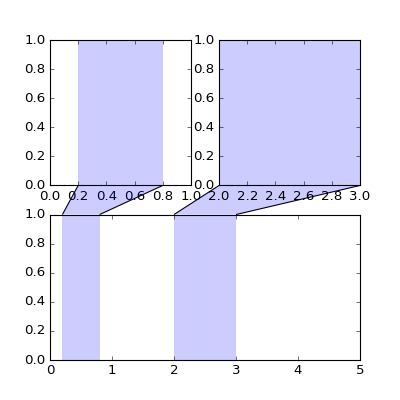## Define Custom BoxStyle¶

You can use a custom box style. The value for the `boxstyle` can be a callable object in following forms.:

```def __call__(self, x0, y0, width, height, mutation_size,
aspect_ratio=1.):
"""
Given the location and size of the box, return the path of
the box around it.

- *x0*, *y0*, *width*, *height* : location and size of the box
- *mutation_size* : a reference scale for the mutation.
- *aspect_ratio* : aspect-ration for the mutation.
"""
path = ...
return path
```

Here is a complete example.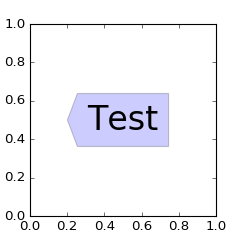However, it is recommended that you derive from the matplotlib.patches.BoxStyle._Base as demonstrated below.

```from matplotlib.path import Path
from matplotlib.patches import BoxStyle
import matplotlib.pyplot as plt

# we may derive from matplotlib.patches.BoxStyle._Base class.
# You need to overide transmute method in this case.

class MyStyle(BoxStyle._Base):
"""
A simple box.
"""

"""
The arguments need to be floating numbers and need to have
default values.

"""

super(MyStyle, self).__init__()

def transmute(self, x0, y0, width, height, mutation_size):
"""
Given the location and size of the box, return the path of
the box around it.

- *x0*, *y0*, *width*, *height* : location and size of the box
- *mutation_size* : a reference scale for the mutation.

Often, the *mutation_size* is the font size of the text.
You don't need to worry about the rotation as it is
automatically taken care of.
"""

width, height = width + 2.*pad, \

# boundary of the padded box
x1, y1 = x0+width, y0 + height

cp = [(x0, y0),
(x1, y0), (x1, y1), (x0, y1),
(x0, y0)]

com = [Path.MOVETO,
Path.LINETO, Path.LINETO, Path.LINETO,
Path.LINETO, Path.LINETO,
Path.CLOSEPOLY]

path = Path(cp, com)

return path

# register the custom style
BoxStyle._style_list["angled"] = MyStyle

plt.figure(1, figsize=(3,3))
ax = plt.subplot(111)
ax.text(0.5, 0.5, "Test", size=30, va="center", ha="center", rotation=30,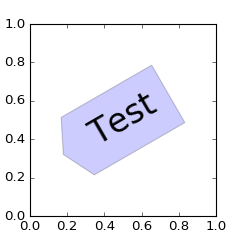Similarly, you can define custom ConnectionStyle and custom ArrowStyle. See the source code of `lib/matplotlib/patches.py` and check how each style class is defined.